# GMAT Math : Understanding powers and roots

## Example Questions

← Previous 1 3 4 5

### Example Question #1 : Powers & Roots Of Numbers

Solve: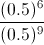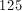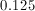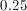Explanation:

Solve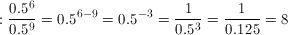### Example Question #1 : Powers & Roots Of Numbers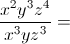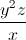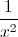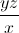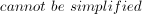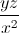Explanation: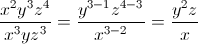### Example Question #3 : Powers & Roots Of Numbers

Solve: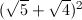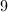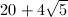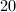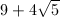Explanation:

First, FOIL: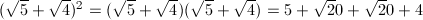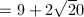Factor out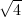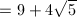### Example Question #2 : Powers & Roots Of Numbers

Solve: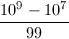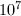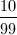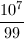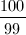Explanation:

First factor.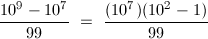Simplify.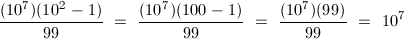### Example Question #5 : Powers & Roots Of Numbers

If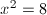, what is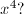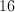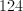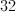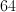Explanation: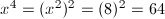### Example Question #6 : Powers & Roots Of Numbers

Evaluate: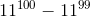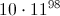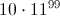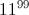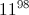Explanation: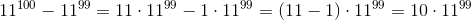### Example Question #7 : Powers & Roots Of Numbers

Simplify this expression as much as possible: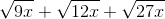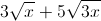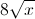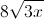The expression cannot be simplified further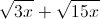Explanation: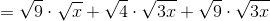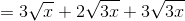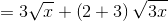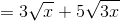### Example Question #8 : Powers & Roots Of Numbers

If the side length of a cube is tripled, how does the volume of the cube change?

Volume becomes 9 times larger.

Volume becomes 3 times larger.

The volume doesn't change.

Not enough informatin is given.

Volume becomes 27 times larger.

Volume becomes 27 times larger.

Explanation:

The equation for the volume of a cube is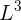.  If the length is tripled, it becomes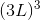, and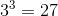, so the volume increases by 27 times the original size.

### Example Question #9 : Powers & Roots Of Numbers

Simplify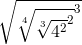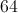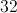Explanation:

This can either be done by brute force (slow) or by recognizing the properties of roots and exponents (fast).  Roots are simply fractional exponents: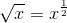,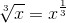, etc. so they can be done in any order.

So we see a cube root, we can immediately cancel that with the exponent of 3. taking us from here:to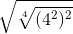.  We now simplify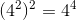to get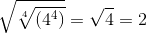### Example Question #10 : Powers & Roots Of Numbers

In the sequence 1, 3, 9, 27, 81, … , each term after the first is three times the previous term. What is the sum of the 9th and 10th terms in the sequence?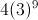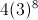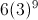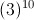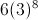Explanation:

We can rewrite the sequence as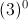,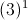,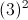,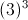,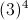, … ,

and we can see that the 9th term in the sequence is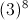and the 10th term in the sequence is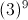. Therefore, the sum of the 9th and 10th terms would be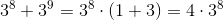← Previous 1 3 4 5

Tired of practice problems?

Try live online GMAT prep today.